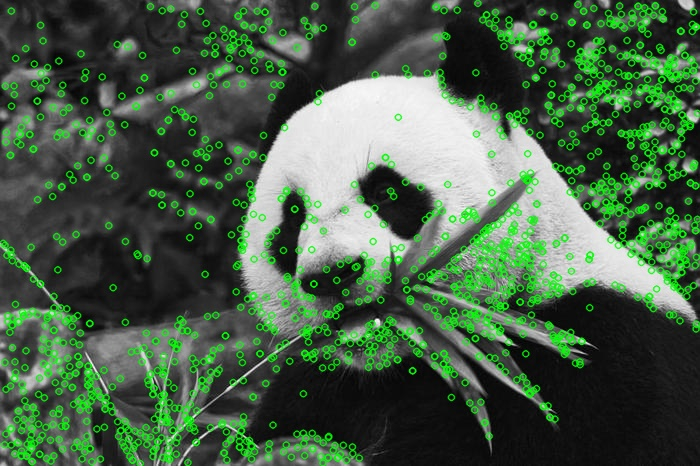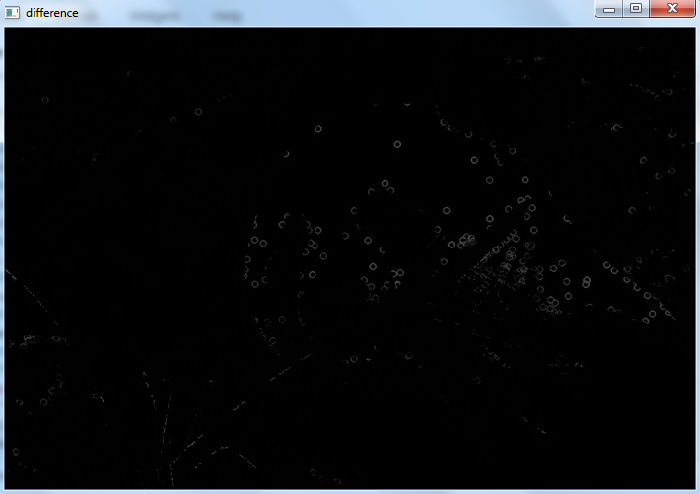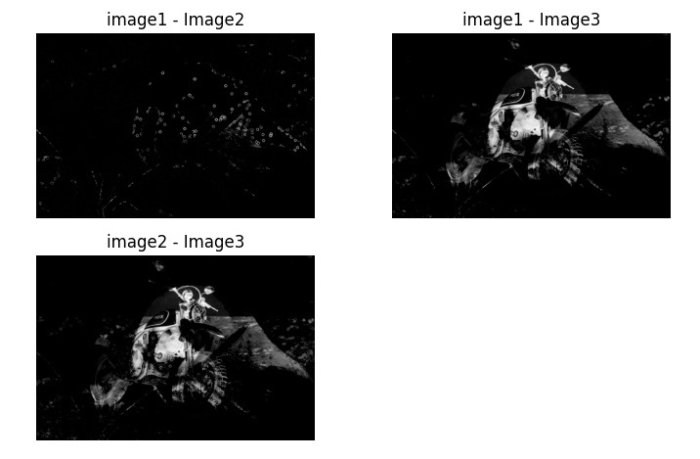# How to compare two images in OpenCV Python?

To compare two images, we use the Mean Square Error (MSE) of the pixel values of the two images. Similar images will have less mean square error value. Using this method, we can compare two images having the same height, width and number of channels.

## Steps

You can use the following steps to compare two images using OpenCV

Import the required library. In all the following Python examples, the required Python library is OpenCV. Make sure you have already installed it.

import cv2


Read the input images using cv2.imread() and convert it to grayscale. The height, width and number of channels of the images must be the same.

img1 = cv2.imread('panda.png')
img1 = cv2.cvtColor(img1, cv2.COLOR_BGR2GRAY)
img2 = cv2.cvtColor(img2, cv2.COLOR_BGR2GRAY)


Define a function to compute the Mean Squared Error between two images.

def mse(img1, img2):
h, w = img1.shape
diff = cv2.subtract(img1, img2)
err = np.sum(diff**2)
mse = err/(float(h*w))
return mse


Compute the Mean Squared Error (Matching error) between the images.

error = mse(img1, img2)


Print the image matching error (mse) and display the image difference.

print("Image matching Error between the two images:", mse)
cv2.imshow("Contour", img)
cv2.waitKey(0)
cv2.destroyAllWindows()


Print the result value, the image shape matching metric. The lower the value, the better matching it is.

print("Matching Image 1 with Image 2:", ret12)


Let's have a look at some examples for a better understanding.

We will use the following images as the Input File in the examples below.## Example 1

In this example, we create a simple Artificial Neural Network with four layers without forward function.

# import required libraries
import cv2
import numpy as np

# convert the images to grayscale
img1 = cv2.cvtColor(img1, cv2.COLOR_BGR2GRAY)
img2 = cv2.cvtColor(img2, cv2.COLOR_BGR2GRAY)

# define the function to compute MSE between two images
def mse(img1, img2):
h, w = img1.shape
diff = cv2.subtract(img1, img2)
err = np.sum(diff**2)
mse = err/(float(h*w))
return mse, diff

error, diff = mse(img1, img2)
print("Image matching Error between the two images:",error)

cv2.imshow("difference", diff)
cv2.waitKey(0)
cv2.destroyAllWindows()


## Output

On execution, it will produce the following output on the console −

Image matching Error between the two images: 3.0696934396076028


And we get the following window showing the difference between the two images −## Example 2

In this Python program, we compare three images.

import cv2
import numpy as np
import matplotlib.pyplot as plt

img1 = cv2.cvtColor(img1, cv2.COLOR_BGR2GRAY)
h, w = img1.shape

img2 = cv2.cvtColor(img2, cv2.COLOR_BGR2GRAY)
img3 = cv2.cvtColor(img3, cv2.COLOR_BGR2GRAY)

def error(img1, img2):
diff = cv2.subtract(img1, img2)
err = np.sum(diff**2)
mse = err/(float(h*w))
msre = np.sqrt(mse)
return mse, diff

match_error12, diff12 = error(img1, img2)
match_error13, diff13 = error(img1, img3)
match_error23, diff23 = error(img2, img3)

print("Image matching Error between image 1 and image 2:",match_error12)
print("Image matching Error between image 1 and image 3:",match_error13)
print("Image matching Error between image 2 and image 3:",match_error23)

plt.subplot(221), plt.imshow(diff12,'gray'),plt.title("image1 - Image2"),plt.axis('off')
plt.subplot(222), plt.imshow(diff13,'gray'),plt.title("image1 - Image3"),plt.axis('off')
plt.subplot(223), plt.imshow(diff23,'gray'),plt.title("image2 - Image3"),plt.axis('off')
plt.show()


## Output

On execution, the above Python program will produce the following output on the console −

Image matching Error between image 1 and image 2: 3.0696934396076028
Image matching Error between image 1 and image 3: 23.37356529736358
Image matching Error between image 2 and image 3: 24.15752299202943


And we get the following window, showing the difference among the images −Notice that the Matching error between the Image1 and Image2 is less in comparison to the matching error between Image 1 & 3 and between Image 2 & 3. So, Image 1 and Image 2 are more similar.

Updated on: 23-Aug-2023

53K+ Views